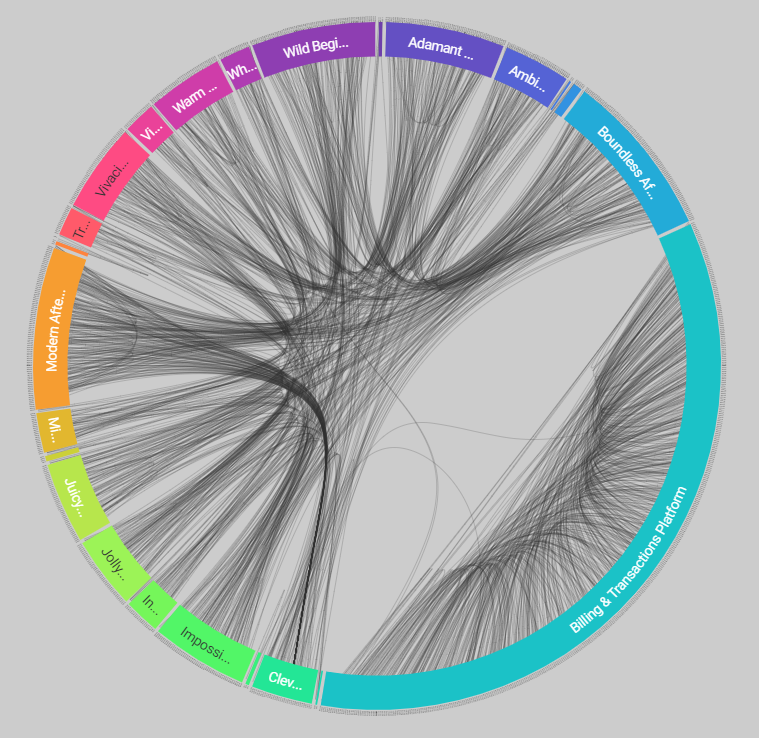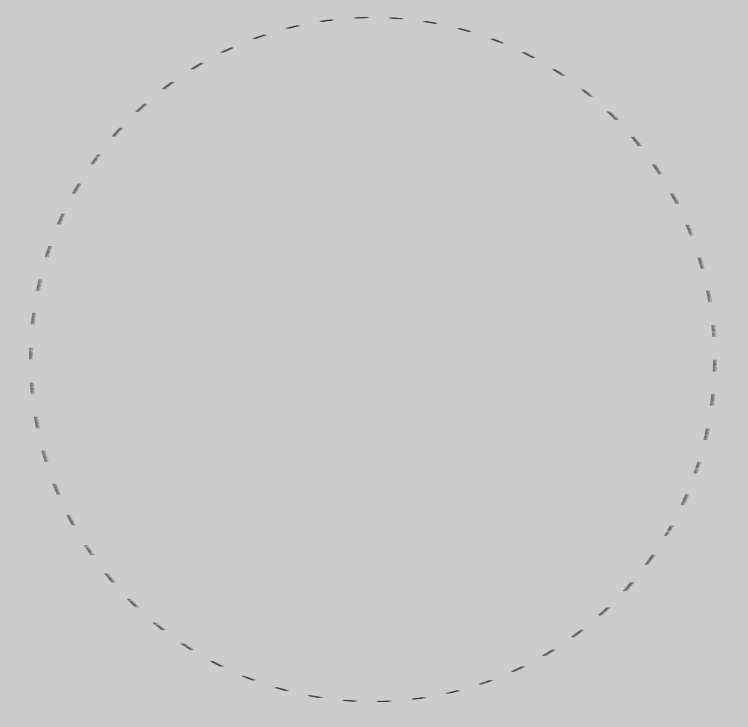# Converting SVG transform to WebGL equivalent

## Recommended Posts

Hi,

I know this isn't the primary use case of PIXI, but I'm trying to convert my SVG data viz, generated primarily with d3.js, to a WebGL equivalent to make it more performant.

The end-result looks like this (svg version), which is essentially a radial dendrogram.Ignoring the coloured arcs for now, I'm stuck trying to convert the nodes (i.e. the black text that is fanned out in a circular rotation) into a WebGL format.

My js is as follows. Just for context I'm looping through an array root.leaves(), which has a d.x value (representing the angle in radians) and a d.y value (representing the radius of the circle).

I create a new PIXI.Text() object for each. I then try to apply the transformations in reverse order of operations, and then add the text to my stage and render it.

I've quickly found out that SVG transformations are quite different to canvas / WebGL equivalents, so any advice here would be appreciated.

``````//original svg code

node = node
.data(root.leaves())
.enter().append("text")
.attr("class", "node")
.attr("id", d => d.data.id)
.attr("transform", d => `rotate(\${d.x * 180 / Math.PI - 90})translate(\${d.y},0)\${d.x >= Math.PI ? `rotate(180)` : ""}`)
.attr("dy", "0.31em")
.attr("x", d => d.x < Math.PI ? 3 : -3)
.attr("font-size", fontValue + "em")
.attr("text-anchor", d => d.x < Math.PI ? "start" : "end")
.text(d => d.data.id)``````
``````//PIXI.js code
root.leaves().forEach(d => {
basicText = new PIXI.Text(d.data.id, {
fontSize: fontValue + "em"
});
basicText.angle = d.x >= Math.PI ? 180 : 0
basicText.x = d.y
basicText.angle = d.x * 180 / Math.PI - 90
//tried using trig functions, almost works?
basicText.x = (width / 2) + (d.y * (Math.cos(d.x * 180 / Math.PI)));
basicText.y = (height / 2) + (d.y * (Math.sin(d.x * 180 / Math.PI)))
//I haven't bothered with the additional dy, x or text-anchor functions until I've nailed the transform part

})

renderer.render(stage);``````

The current output seems like I'm on the right track, but for some reason the text is clustering around discrete points on the circle instead of a uniform distribution.

FYI I'm using v4 of PIXI for now.##### Share on other sites

OMG. That is not possible without shader tricks to make thin materials, something like what https://twitter.com/FreyaHolmer uses for Unity plugin. As for text, you defenitely need to look inside Text sources and modify it. maybe even take text from our experimental branch https://github.com/pixijs/pixi.js/pull/6597 Maybe take one of old sdf plugins and convert it to v5, i dont remember if someone changed pixi-sdf-text.

One of easiest things you can do is to use Rope on text texture.

##### Share on other sites

Oh, no, you wont hide that oneI want to reference it when we finally go for thin lines and demos for curved text.

Edited by ivan.popelyshev

## Join the conversation

You can post now and register later. If you have an account, sign in now to post with your account.
Note: Your post will require moderator approval before it will be visible.×   Pasted as rich text.   Paste as plain text instead

Only 75 emoji are allowed.